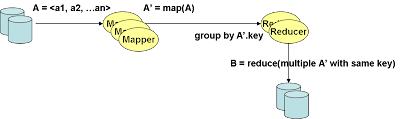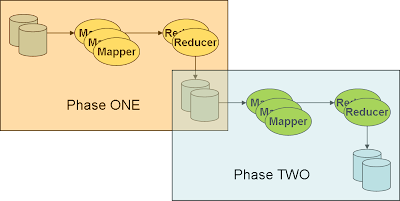## Sunday, May 25, 2008

### Parallel data processing language for Map/Reduce

In my previous post, I introduce Map/Reduce model as a powerful model for parallelism. However, although Map/Reduce is simple, powerful and provide a good opportunity to parallelize algorithm, it is based on a rigid procedural structure that require injection of custom user code and therefore it not easy to understand the big picture from a high level. You need to drill into the implementation code of the map and reduce function in order to figure out what is going on.

It will be desirable to have a higher level declarative language that describe the parallel data processing model. This is similar to the idea of SQL query where the user specify the "what" and leave the "how" to the underlying processing engine. In this post, we will explore the possibility of such a declarative language. We will start from the Map/Reduce model and see how it can be generalized into a "Parallel data processing model".

Lets revisit Map/Reduce in a more abstract sense.The Map/Reduce processing model composes of the following steps ...
• From many distributed data store, InputReader extract out data tuples A = <a1,a2,...> and feed them randomly into the many Map tasks.
• For each tuple A, the Map task emit zero to many tuples A'
• The output A' will be sorted by its key, A' with the same key will reach the same Reduce task
• The Reduce task aggregate over the group of tuples A' (of the same key) and then turn them into a tuple B = reduce(array<A'>)
• The OutputWriter store the data tuple B into the distributed data store.
Paralleizing more sophisticated algorithm typically involve multiple phases of Map/Reduce phases, each phase may have a different Map task and Reduce task.Looking at the abstract Map/Reduce model, there are some similarities with the SQL query model. We can express the above Map/Reduce model using a SQL-like query language.

``````INSERT INTO A FROM InputReader("dfs:/data/myInput")

INSERT INTO A'
SELECT flatten(map(*)) FROM A

INSERT INTO B
SELECT reduce(*) FROM A' GROUP BY A'.key

INSERT INTO  "dfs:/data/myOutput"  FROM B``````

Similarly, SQL queries can also be expressed by different forms of map() and reduce() functions. Lets look at a couple typical SQL query examples.

Simple Query
``````SELECT a1, a2 FROM A
WHERE a3 > 5 AND a4 < 6``````

Here is the corresponding Map and Reduce function
``````def map(tuple)
/* tuple is implemented as a map, key by attribute name */
if  (tuple["a3"] > 5  &&  tuple["a4"] < 6)
key = random()
emit key, "a1" => tuple["a1"], "a2" => tuple["a2"]
end
end

def reduce(tuples)
tuples.each do |tuple|
store tuple
end
end``````

Query with Grouping
``````SELECT sum(a1), avg(a2) FROM A
GROUP BY a3, a4
HAVING count() < 10
``````
Here is the coresponding Map and Reduce function
``````def map(tuple)
key = [tuple["a3"], tuple["a4"]]
emit key, "a1" => tuple["a1"], "a2" => tuple["a2"]
end

def reduce(tuples)
sums = {"a1" => 0, "a2" => 0}
count = 0

tuples.each do |tuple|
count += 1
sums.each_key do |attr|
sums[attr] += tuple[attr]
end
end

if count < 10
/* omit denominator check for simplcity */
store {"type" => B, "b1" => sums["a1"], "b2" => sums["a2"] / count}
end
end``````

Query with Join
``````SELECT a2, p2
FROM A JOIN P
ON A.a1 = P.p1``````
Here is the corresponding Map and Reduce function
``````def map(tuple)
if (tuple["type"] == A)
key = tuple["a1"]
emit key, "a2" => tuple["a2"]
elsif (tuple["type"] == P)
key = tuple["p1"]
emit key, "p2" => tuple["p2"]
end
end

def reduce(tuples)
all_A_tuples = []
all_P_tuples = []

tuples.each do |tuple|
if (tuple["type"] == A)
all_P_tuples.each do |p_tuple|
joined_tuple = p_tuple.merge(tuple)
joined_tuple["type"] = B
store joined_tuple
end
elsif (tuple["type"] == P)
/* do similar things */
end
end
end``````

As you can see, transforming a SQL query to Map/Reduce function is pretty straightforward.

We put the following logic inside the map() function
• Select columns that appears in the SELECT clause
• Evaluate the WHERE clause and filter out tuples that doesn't match the condition
• Compute the key for the JOIN clause or the GROUP clause
• Emit the tuple

On the other hand, we put the following logic inside the reduce() function
• Compute the aggregate value of the columns appears in the SELECT clause
• Evaluate the HAVING clause and filter things out
• Compute the cartesian product of the JOIN clause
• Store the final tuple
As we've seen the potential opportunity to use a "SQL-like" declarative language to express the parallel data processing and use a Map/Reduce model to execute it, the open source Hadoop community is working on a project call Pig to develop such a language.

PIG is similar to SQL in the following way.
• PIG's tuple is same as SQL record, containing multiple fields
• PIG has define its own set
• Like SQL optimizer which compiles the query into an execution plan, PIG compiler compiles its query into a Map/Reduce task.

However, there are a number of important difference between PIG (in its current form) and the SQL language.
• While fields within a SQL record must be atomic (contain one single value), fields within a PIG tuple can be multi-valued, e.g. a collection of another PIG tuples, or a map with key be an atomic data and value be anything
• Unlike relational model where each DB record must have a unique combination of data fields, PIG tuple doesn't require uniqueness.
• Unlike SQL query where the input data need to be physically loaded into the DB tables, PIG extract the data from its original data sources directly during execution.
• PIG is lazily executed. It use a backtracking mechansim from its "store" statement to determine which statement needs to be executed.
• PIG is procedural and SQL is declarative. In fact, PIG looks a lot like a SQL query execution plan.
• PIG enable easy plug-in of user defined functions
For more details, please refer to PIG's project site.